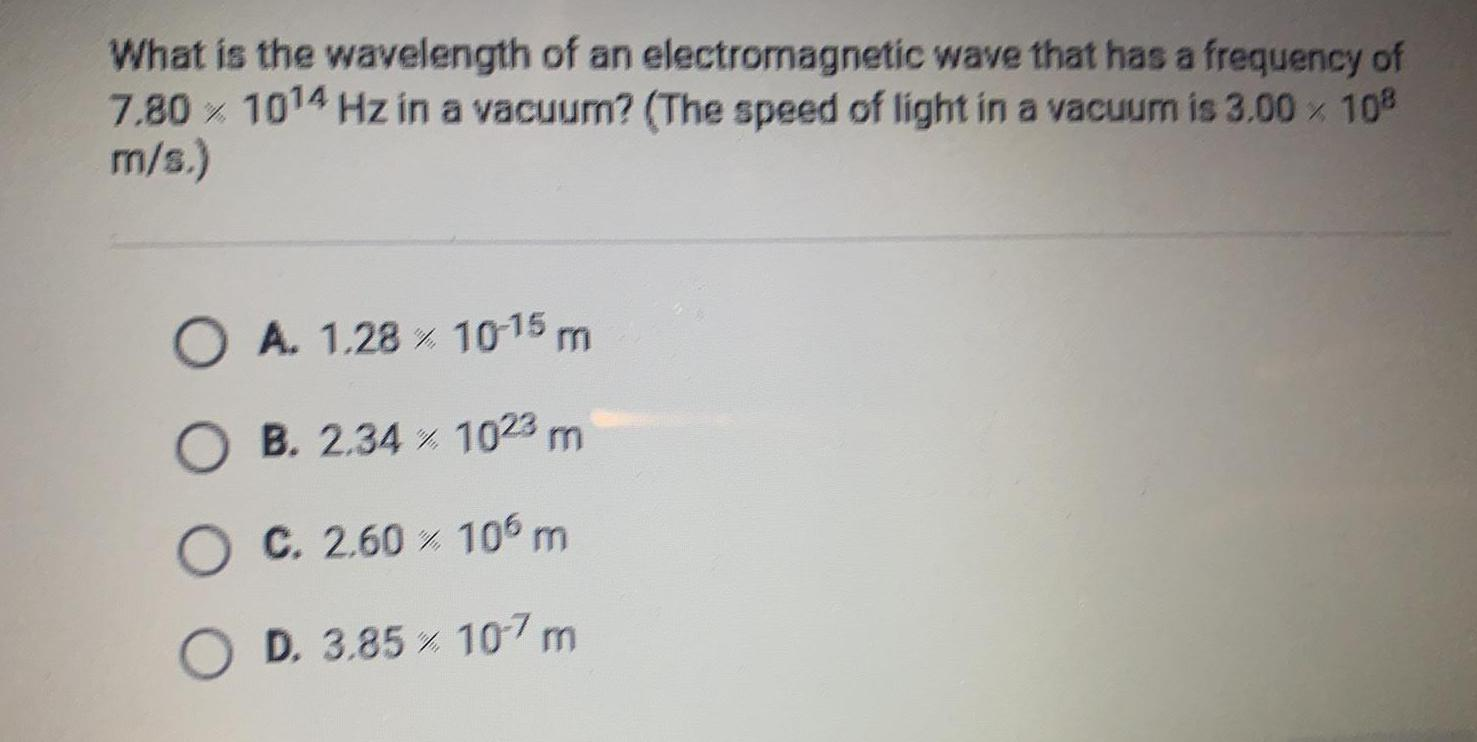Question:

# What is the wavelength of an electromagnetic wave that has a

Last updated: 7/31/2022What is the wavelength of an electromagnetic wave that has a frequency of 7.80 x 1014 Hz in a vacuum? (The speed of light in a vacuum is 3.00 × 108 m/s.) A. 1.28% 10^-15 m B. 2.34% 10^23 m C. 2.60% 10^6 m D. 3.85 x 10^-7 m